×#### Thank you for registering.

One of our academic counsellors will contact you within 1 working day.

Click to Chat

1800-1023-196

+91-120-4616500

CART 0

• 0

MY CART (5)

Use Coupon: CART20 and get 20% off on all online Study Material

ITEM
DETAILS
MRP
DISCOUNT
FINAL PRICE
Total Price: Rs.

There are no items in this cart.
Continue Shopping• Complete JEE Main/Advanced Course and Test Series
• OFFERED PRICE: Rs. 15,900
• View Details

Chapter 8: Linear Equations in One Variable Exercise – 8.2

Question: 1

x – 3 = 5

Solution:

x – 3 = 5

Adding 3 to both sides, we get

x – 3 + 3 = 5 + 3

x = 8

Verification:

Substituting x = 8 in LHS, we get

LHS = x – 3 and RHS = 5

LHS = 8 – 3 = 5 and RHS = 5

LHS = RHS

Hence, verified.

Question: 2

x + 9 = 13

Solution:

x + 9 = 13

Subtracting 9 from both sides, we get

=> x + 9 – 9 = 13 – 9

=> x = 4

Verification:

Substituting x = 4 on LHS, we get

LHS = 4 + 9 = 13 = RHS

LHS = RHS

Hence, verified.

Question: 3Solution:Question: 4

3x = 0

Solution:

3x = 0

Dividing both sides by 3, we get

3x/3 = 0/3

x = 0

Verification:

Substituting x = 0 in LHS = 3x, we get LHS = 3 × 0 = 0 and RHS = 0

LHS = RHS

Hence, verified.

Question: 5

x/2 = 0

Solution:

x/2 = 0

Multiplying both sides by 2, we get

=> (x/2) × 2 = 0 × 2

=> x = 0

Verification:

Substituting x = 0 in LHS, we get

LHS = 0/2 = 0 and RHS = 0

LHS = 0 and RHS = 0

LHS = RHS

Question: 6Solution: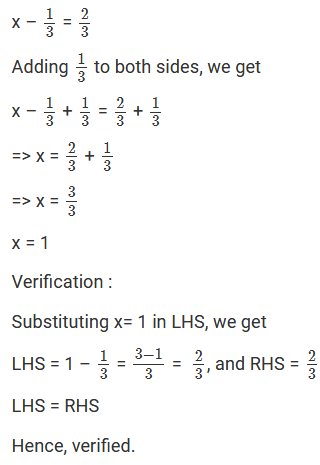Question: 7Solution:Question: 8

10 – y = 6

Solution:

10 – y = 6

Subtracting 10 from both sides, we get

10 – y – 10 = 6 – 10

-y = -4

Multiplying both sides by -1, we get

-y × -1 = - 4 × - 1

y = 4

Verification:

Substituting y = 4 in LHS, we get

LHS = 10 – y = 10 – 4 = 6 and RHS = 6

LHS = RHS

Hence, verified.

Question: 9

7 + 4y = - 5

Solution:

7 + 4y = -5

Subtracting 7 from both sides, we get

7 + 4y – 7 = -5 -7

4y = -12

Dividing both sides by 4, we get

y = -12/ 4

y = -3

Verification:

Substituting y = -3 in LHS, we get

LHS = 7 + 4y = 7 + 4(-3) = 7 – 12 = -5, and RHS = -5

LHS = RHS

Hence, verified.

Question: 10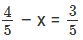Solution: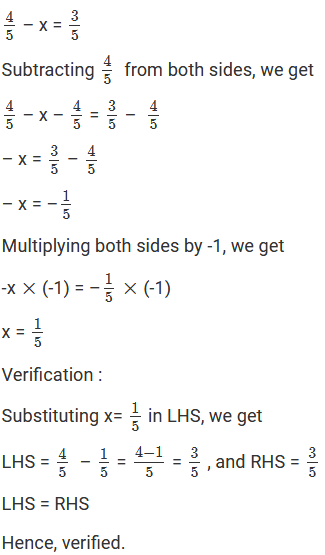Question: 11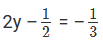Solution:Question: 12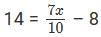Solution:Adding 8 to both sides, we get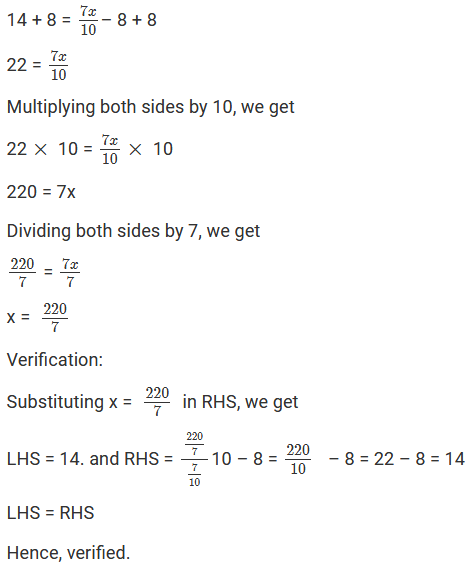Question: 13

3(x + 2) = 15

Solution:

3 (x + 2) = 15

Dividing both sides by 3, we get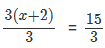(x + 2) = 5

Subtracting 2 from both sides, we get

x + 2 – 2 = 5 – 2

x = 3

Verification:

Substituting x = 3 in LHS, we get

LHS = 3 (x + 2) = 3 (3 + 2) = 3(5) = 15, and RHS = 15

LHS = RHS

Hence, verified.

Question: 14

x/4 = 7/8

Solution:

x/4 = 7/8

Multiplying both sides by 4, we getQuestion: 15

1/3 - 2x = 0

Solution:

1/3 - 2x = 0

Subtracting 13 from both sides, we get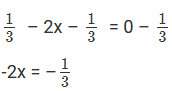Multiplying both sides by -1, we getLHS = RHS

Hence, verified.

Question: 16

3(x + 6) = 24

Solution:

3(x + 6) = 24

Dividing both sides by 3, we get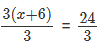(x + 6) = 8

Subtracting 6 from both sides, we get

x + 6 – 6 = 8 – 6

x = 2

Verification:

Substituting x = 2 in LHS, we get

LHS = 3(x + 6) = 3(2 + 6) = 24, and RHS = 24

LHS = RHS

Hence, verified.

Question: 17

3(x + 2) - 2(x – 1) = 7

Solution:

3(x + 2) – 2(x – 1) = 7

On expanding the brackets, we get

3× x + 3 × 2 – 2 × x + 2 × 1 = 7

3x + 6 – 2x + 2 = 7

3x – 2x + 6 + 2 = 7

x + 8 = 7

Subtracting 8 from both sides, we get

x + 8 – 8 = 7 – 8

x = -1

Verification:

Substituting x = -1 in LHS, we get

LHS = 3 (x + 2) -2(x -1) = 3 (-1 + 2) -2(-1-1) = (3×1) – (2×-2) = 3 + 4 = 7, and RHS = 7

LHS = RHS

Hence, verified.

Question: 18

8(2x – 5) - 6(3x – 7) = 1

Solution:

8(2x – 5) – 6(3x – 7) = 1

On expanding the brackets, we get (8 × 2x) – (8 × 5) – (6 × 3x) + (-6) × (-7) = 1

16x – 40 – 18x + 42 = 1

16x – 18x + 42 – 40 = 1

-2x + 2 = 1

Subtracting 2 from both sides, we get

-2x+ 2 – 2 = 1 -2

-2x = -1

Multiplying both sides by -1, we get

-2x × (-1) = -1× (-1)

2x = 1

Dividing both sides by 2, we get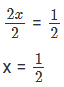Verification:

Substituting x = 1/2 in LHS, we get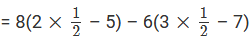= 8(1 – 5) – 6(32 – 7)

= 8× (-4) – (6 × 32) + (6 × 7) = -32 – 9 + 42 = – 41 + 42 = 1= RHS

LHS = RHS

Hence, verified.

Question: 19

6(1 – 4x) + 7(2 + 5x) = 53

Solution:

6(1 – 4x) + 7(2 + 5x) = 53

On expanding the brackets, we get (6 ×1) – (6 × 4x) + (7 × 2) + (7 × 5x) = 53

6 – 24x + 14 + 35x = 53

6 + 14 + 35x – 24x = 53

20 + 11x = 53

Subtracting 20 from both sides, we get 20 + 11x – 20 = 53 – 20

11x = 33

Dividing both sides by 11, we get

11x/11 = 33/11

x = 3

Verification:

Substituting x = 3 in LHS, we get

= 6(1 – 4 × 3) + 7(2 + 5 × 3)

= 6(1 – 12) + 7(2 + 15)

= 6(-11) + 7(17)

= - 66 + 119

= 53 = RHS

LHS = RHS

Hence, verified.

Question: 20

5(2 – 3x) - 17(2x – 5) = 16

Solution:

5(2 – 3x) -17(2x – 5) = 16

On expanding the brackets, we get (5 × 2) – (5 × 3x) – (17 × 2x) + (17 × 5) = 16

10 – 15x – 34x + 85 = 16

10 + 85 – 34x – 15x = 16

95 – 49x = 16

Subtracting 95 from both sides, we get – 49x + 95 – 95 = 16 – 95

-49x = -79

Dividing both sides by – 49, we get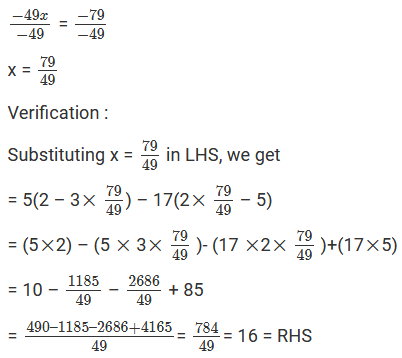LHS = RHS

Hence, verified.

Question: 21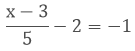Solution:Adding 2 to both sides, we get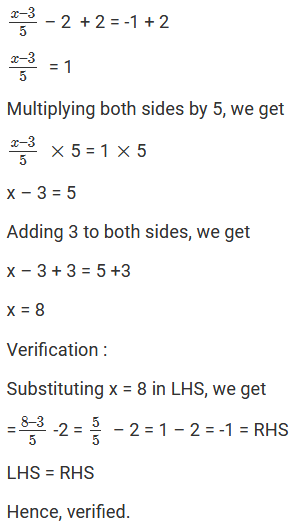Question: 22

5(x – 2) +3(x + 1) = 25

Solution:

5(x – 2) + 3(x + 1) = 25

On expanding the brackets, we get

(5 × x) – (5 × 2) +3×  x + 3× 1 = 25

5x – 10 + 3x + 3 = 25

5x + 3x – 10 + 3 = 25

8x – 7 = 25

Adding 7 to both sides, we get

8x – 7 + 7 = 25 + 7

8x = 32

Dividing both sides by 8, we get

8x/8 = 32/8

x = 4

Verification :

Substituting x = 4 in LHS, we get

= 5(4 – 2) + 3(4 + 1) = 5(2) + 3(5) = 10 + 15 = 25 = RHS

LHS = RHS

Hence, verified.### Course Features

• 728 Video Lectures
• Revision Notes
• Previous Year Papers
• Mind Map
• Study Planner
• NCERT Solutions
• Discussion Forum
• Test paper with Video Solution